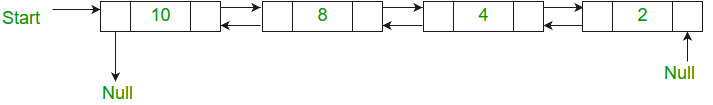GeeksforGeeks App
Open AppBrowser
Continue

# Python Program For Merge Sort For Doubly Linked List

Given a doubly linked list, write a function to sort the doubly linked list in increasing order using merge sort.
For example, the following doubly linked list should be changed to 24810Merge sort for singly linked list is already discussed. The important change here is to modify the previous pointers also when merging two lists.

Below is the implementation of merge sort for doubly linked list.

## Python

 `# Program for merge sort on doubly linked list``# A node of the doubly linked list``class` `Node:``     ` `    ``# Constructor to create a new node``    ``def` `__init__(``self``, data):``        ``self``.data ``=` `data ``        ``self``.``next` `=` `None``        ``self``.prev ``=` `None`` ` `class` `DoublyLinkedList:`` ` `     ``# Constructor for empty Doubly ``     ``# Linked List``    ``def` `__init__(``self``):``        ``self``.head ``=` `None`` ` `    ``# Function to merge two linked list``    ``def` `merge(``self``, first, second):``         ` `        ``# If first linked list is empty``        ``if` `first ``is` `None``:``            ``return` `second ``         ` `        ``# If second linked list is empty ``        ``if` `second ``is` `None``:``            ``return` `first`` ` `        ``# Pick the smaller value``        ``if` `first.data < second.data:``            ``first.``next` `=` `self``.merge(first.``next``, ``                                    ``second)``            ``first.``next``.prev ``=` `first``            ``first.prev ``=` `None`   `            ``return` `first``        ``else``:``            ``second.``next` `=` `self``.merge(first, ``                                     ``second.``next``)``            ``second.``next``.prev ``=` `second``            ``second.prev ``=` `None``            ``return` `second`` ` `    ``# Function to do merge sort``    ``def` `mergeSort(``self``, tempHead):``        ``if` `tempHead ``is` `None``: ``            ``return` `tempHead``        ``if` `tempHead.``next` `is` `None``:``            ``return` `tempHead``         ` `        ``second ``=` `self``.split(tempHead)``         ` `        ``# Recur for left and right halves``        ``tempHead ``=` `self``.mergeSort(tempHead)``        ``second ``=` `self``.mergeSort(second)`` ` `        ``# Merge the two sorted halves``        ``return` `self``.merge(tempHead, second)`` ` `    ``# Split the doubly linked list (DLL) into ``    ``# two DLLs of half sizes``    ``def` `split(``self``, tempHead):``        ``fast ``=` `slow ``=`  `tempHead``        ``while``(``True``):``            ``if` `fast.``next` `is` `None``:``                ``break``            ``if` `fast.``next``.``next` `is` `None``:``                ``break``            ``fast ``=` `fast.``next``.``next` `            ``slow ``=` `slow.``next``             ` `        ``temp ``=` `slow.``next``        ``slow.``next` `=` `None``        ``return` `temp``         ` `             ` `    ``# Given a reference to the head of a list and an``    ``# integer,inserts a new node on the front of list``    ``def` `push(``self``, new_data):``  ` `        ``# 1. Allocates node``        ``# 2. Put the data in it``        ``new_node ``=` `Node(new_data)``  ` `        ``# 3. Make next of new node as head and``        ``# previous as None (already None)``        ``new_node.``next` `=` `self``.head``  ` `        ``# 4. change prev of head node to new_node``        ``if` `self``.head ``is` `not` `None``:``            ``self``.head.prev ``=` `new_node``  ` `        ``# 5. move the head to point to the new node``        ``self``.head ``=` `new_node`` ` ` ` `    ``def` `printList(``self``, node):``        ``temp ``=` `node``        ``print` `"Forward Traversal using next pointer"``        ``while``(node ``is` `not` `None``):``            ``print` `node.data,``            ``temp ``=` `node``            ``node ``=` `node.``next``        ``print` `"``Backward Traversal using prev pointer"``        ``while``(temp):``            ``print` `temp.data,``            ``temp ``=` `temp.prev`` ` `# Driver program to test the above functions``dll ``=` `DoublyLinkedList()``dll.push(``5``)``dll.push(``20``);``dll.push(``4``);``dll.push(``3``);``dll.push(``30``)``dll.push(``10``);``dll.head ``=` `dll.mergeSort(dll.head)   ``print` `"Linked List after sorting"``dll.printList(dll.head)`` ` `# This code is contributed by Nikhil Kumar Singh(nickzuck_007)`

Output:

```Linked List after sorting
Forward Traversal using next pointer
3 4 5 10 20 30
Backward Traversal using prev pointer
30 20 10 5 4 3```

Time Complexity: Time complexity of the above implementation is same as time complexity of MergeSort for arrays. It takes Θ(nLogn) time.

Space Complexity:O(1). We are only using constant amount of extra space.
You may also like to see QuickSort for doubly linked list
Please refer complete article on Merge Sort for Doubly Linked List for more details!

My Personal Notes arrow_drop_up